We’d like to understand how you use our websites in order to improve them. Register your interest.

# On the solvability of the boundary value problem without initial condition for Schrödinger systems in infinite cylinders

## Abstract

In this paper, we deal with the boundary value problems without initial condition for Schrödinger systems in cylinders. We establish several results on the existence and uniqueness of solutions.

## Introduction

The initial boundary value for the Schrödinger equation in cylinders with base containing conical points was established in . Such a problem for parabolic systems was studied in Sobolev spaces with weights . The boundary value problem without initial condition for parabolic equation was investigated in .

In the present paper, we consider the boundary value problem without initial condition for Schrödinger systems in cylinders. Firstly, following the method in , we prove the existence of solutions $u h$ of problems with initial conditions $t=h$. Then, by letting $h→−∞$, the solvability of a problem without initial condition is obtained.

This paper is organized as follows. In the first section, we state the problem. In Section 2, we present the results on the unique solvability of problems with initial condition for Schrödinger systems in cylinders. The well-posedness of the problem without initial condition is dealt with in Sections 3 and 4.

## 1 Setting the problem

Let Ω be a bounded domain in $R n$ ($n≥2$) with boundary $S=∂Ω$. For $a, set $Ω a b =Ω×(a,b)$, $S a b =S×(a,b)$. If $(a,b)=R$, we use $Ω R$ to refer to $Ω − ∞ ∞$ and $S R$ to refer to $S − ∞ ∞$. For each multi-index $α=( α 1 ,…, α n )∈ N n$, set $|α|= α 1 +⋯+ α n$ and $∂ α = ∂ x 1 α 1 ⋯ ∂ x n α n$.

Denote $u(x,t)=( u 1 (x,t),…, u s (x,t))$, $D α u=( D α u 1 ,…, D α u s )$, $| D α u | 2 = ∑ i = 1 s | D α u i | 2$ and $u t j =( ∂ j u 1 ∂ t j ,…, ∂ j u s ∂ t j )$ , $| u t j | 2 = ∑ i = 1 s | ∂ j u i ∂ t j | 2$.

Let us introduce some functional spaces (see ) used in this paper.

We use $H k (Ω)$ to be the space of s-dimensional vector functions defined in Ω with the norm

$∥ u ∥ H k ( Ω ) = ( ∑ | α | = 0 k ∫ Ω | D α u | 2 d x ) 1 2 .$

Denote by $H k , l ( Ω a b )$ the space consisting of all vector functions $u: Ω a b ⟶ C s$ satisfying

$∥ u ∥ H k , l ( Ω a b ) = ( ∫ Ω a b ∑ | α | = 0 k | D α u | 2 + ∑ j = 1 l | u t j | 2 d x d t ) 1 2 ,$

and $H k , l (−γ, Ω a b )$ is the space of vector functions with the norm

$∥ u ∥ H k , l ( − γ , Ω a b ) = ( ∫ Ω a b ( ∑ | α | = 0 k | D α u | 2 + ∑ j = 1 l | u t j | 2 ) e − 2 γ t d x d t ) 1 2 .$

In particular,

$∥ u ∥ H k , 0 ( − γ , Ω a b ) = ( ∑ | α | = 0 k ∫ Ω a b | D α u | 2 e − 2 γ t d x d t ) 1 2 .$

Especially, we set $L 2 (−γ, Ω a b )= H 0 , 0 (−γ, Ω a b )$.

Denote by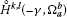the completion of infinitely differentiable vector functions vanishing near $S a b$ with respect to $H l , k (−γ, Ω a b )$ norm.

Now we introduce a differential operator of order 2m

$L(x,t,D)= ∑ | p | , | q | = 0 m ( − 1 ) | p | D p ( a p q ( x , t ) D q ) ,$

where $a p q$ are $s×s$ matrices with the bounded complex-valued components in $Ω ¯ R$, $a p q = a q p ∗$ ($a q p ∗$ is the transposed conjugate matrix to $a p q$). Set

$B(t,u,v)= ∑ | p | , | q | = 0 m ∫ Ω a p q D q u D p v ¯ dx,t∈R.$

We assume further that the form $B(t,⋅,⋅)$ is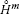-elliptic uniformly with respect to $t∈R$, which means there exists a constant $μ 0 >0$ independent of t and u such that

$B(t,u,u)≥ μ 0 ∥ u ( ⋅ , t ) ∥ H m ( Ω ) 2$
(1.1)

for all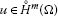and a.e. $t∈R$, where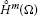is a subspace of $H m (Ω)$, the dense subset of infinitely differentiable complex s-dimensional vector functions with compact support in Ω.

Now we consider the following problem in the cylinder $Ω R$:

(1.2)
$∂ j u ∂ ν j | S R =0,j=0,…,m−1,$
(1.3)

where ν is the unit vector of outer normal to the surrounding surface $S R$.

Let $f∈ L 2 (−γ, Ω R )$, a complex vector-valued functionis called a generalized solution of problem (1.2)-(1.3) if and only if, for any $T>0$, the equality

$( − 1 ) m − 1 i ∫ − ∞ T B(t,u,η)dt+ ∫ Ω − ∞ T u η t ¯ dxdt= ∫ Ω − ∞ T f η ¯ dxdt$
(1.4)

holds for all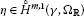, $η(x,t)=0$ with $t≥T$.

## 2 The unique solvability of problems with initial condition

Firstly, for any $h∈R$, we study the following problem in the cylinder $Ω h ∞$:

(2.1)
$v ∣ t = h =0,x∈Ω,$
(2.2)
$∂ j v ∂ ν j | S h ∞ =0,j=0,…,m−1,$
(2.3)

where ν is the unit vector of outer normal to the surrounding surface $S h ∞$.

The solution $v(x,t)$ is surveyed in the generalized sense. That means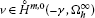is a generalized solution if and only if, for any $T>0$, we have

$( − 1 ) m − 1 i ∫ h T B(t,v,η)dt+ ∫ Ω h T v η t ¯ dxdt= ∫ Ω h T f η ¯ dxdt$
(2.4)

for all, $η(x,t)=0$ for all $t≥T$.

In , the unique solvability of problem (2.1)-(2.3) is studied in the case $h=0$ and $f, f t ∈ L ∞ (0,∞; L 2 (Ω))$. Now, by the same method, we consider that problem in the case $h∈R$ and $f, f t ∈ L 2 (−γ, Ω h ∞ )$.

Theorem 2.1 Assume that

1. (i)

$sup{| ∂ a p q ∂ t |:(x,t)∈ Ω h ∞ ,0≤|p|,|q|≤m}=μ<∞$;

2. (ii)

$f t ,f∈ L 2 (−γ, Ω h ∞ )$.

Then, for all $γ> γ 0 = m ⋆ μ 2 μ 0$, $m ⋆ = ∑ | α | ≤ m 1$, there exists a uniquely generalized solutionof problem (2.1)-(2.3) satisfying

$∥ v ∥ H m , 0 ( − γ , Ω h ∞ ) 2 ≤C [ ∥ f ∥ L 2 ( − γ , Ω h ∞ ) 2 + ∥ f t ∥ L 2 ( − γ , Ω h ∞ ) 2 ] ,$
(2.5)

where C is a nonnegative constant independent of h, v, and f.

Proof The uniqueness is proved in a similar way as in . We omit the details here. Now we prove the existence by the Galerkin approximating method. Suppose that ${ φ k } k = 1 ∞$ is an orthogonal basis of $H m (Ω)$ which is orthonormal in $L 2 (Ω)$. For any $N∈N$, we consider the function $v N (x,t)= ∑ k = 1 N C k N (t) φ k (x)$, where $( C k N ( t ) ) k = 1 N$ is the solution of the ordinary differential system

$( − 1 ) m − 1 i B ( t , v N , φ k ) − ∫ Ω v t N φ k ¯ d x = ∫ Ω f φ k ¯ d x , C k N ( h ) = 0 , k = 1 , … , N .$
(2.6)

So, multiplying both sides of (2.6) by $d d t ( C k N ( t ) ) ¯$ and taking the sum with respect to k from 1 to N, we arrive at

$( − 1 ) m ∑ | p | , | q | = 0 m ∫ Ω a p q D q v N D p v t N ¯ dx−i ∫ Ω v t N v t N ¯ dx=i ∫ Ω f v t N ¯ dx.$

Adding this equation to its complex conjugate, integrating with respect to t from h to T, and then integrating by parts, we get

$( − 1 ) m ∑ | p | , | q | = 0 m ∫ Ω a p q ( x , T ) D q v N ( x , T ) D p v N ( x , T ) ¯ d x = ( − 1 ) m ∑ | p | , | q | = 0 m ∫ Ω h T ∂ a p q ∂ t D q v N D p v N ¯ d x d t − 2 Im ( ∫ Ω f ( x , T ) v N ( x , T ) ¯ d x − ∫ Ω h T f t v N ¯ d x d t ) .$
(2.7)

Using (1.1) and the Cauchy inequality, we receive from (2.7) that

$∥ v N ( ⋅ , T ) ∥ H m ( Ω ) 2 ≤ m ⋆ μ + ϵ μ 0 − ϵ ∫ h T ∥ v N ( ⋅ , t ) ∥ H m ( Ω ) 2 d t + 1 ϵ ( μ 0 − ϵ ) ( ∥ f ( ⋅ , T ) ∥ L 2 ( Ω ) 2 + ∫ h T ∥ f t ( ⋅ , t ) ∥ L 2 ( Ω ) 2 d t ) .$
(2.8)

Using the Gronwall-Bellman inequality, put $2α= m ⋆ μ + ϵ μ 0 − ϵ$, from (2.8) we obtain

$∥ v N ( ⋅ , T ) ∥ H m ( Ω ) 2 ≤ 2 α ∫ h T e 2 α ( T − t ) ( ∥ f ( ⋅ , t ) ∥ L 2 ( Ω ) 2 + ∫ h T ∥ f s ( ⋅ , s ) ∥ L 2 ( Ω ) 2 d s ) d t + 1 ϵ ( μ 0 − ϵ ) ( ∥ f ( ⋅ , T ) ∥ L 2 ( Ω ) 2 + ∫ h T ∥ f t ( ⋅ , t ) ∥ L 2 ( Ω ) 2 d t ) .$

Multiplying both sides of this equation by $e − 2 γ T$ and integrating with respect to T from h to ∞, we get

$∥ v N ∥ H m , 0 ( − γ , Ω h ∞ ) 2 ≤ 1 ϵ ( μ 0 − ϵ ) ∫ h ∞ e − 2 γ T ∥ f ( ⋅ , T ) ∥ L 2 ( Ω ) 2 d T + 1 ϵ ( μ 0 − ϵ ) ∫ h ∞ e − 2 γ T ∫ h T ∥ f t ( ⋅ , t ) ∥ L 2 ( Ω ) 2 d t d T + 2 α ∫ h ∞ e − 2 γ T ∫ h T e 2 α ( T − t ) ∥ f ( ⋅ , t ) ∥ L 2 ( Ω ) 2 d t d T + 2 α ∫ h ∞ e − 2 γ T ∫ h T e 2 α ( T − t ) ∫ h t ∥ f s ( ⋅ , s ) ∥ L 2 ( Ω ) 2 d s d t d T .$
(2.9)

We denote by I, II, III, IV the terms from the first, second, third, fourth, respectively, of the right-hand sides of (2.9). We will give estimations for these terms. Firstly

$I= 1 ϵ ( μ 0 − ϵ ) ∥ f ∥ L 2 ( − γ , Ω h ∞ ) 2 ,$

and

$II= 1 ϵ ( μ 0 − ϵ ) ∫ h ∞ ∥ f t ( ⋅ , t ) ∥ L 2 ( Ω ) 2 ∫ t ∞ e − 2 γ T dTdt= 1 2 γ ϵ ( μ 0 − ϵ ) ∥ f t ∥ L 2 ( − γ , Ω h ∞ ) 2 .$

Because of $inf 0 < ϵ < μ 0 m ⋆ μ + ϵ μ 0 − ϵ = m ⋆ μ μ 0 =2 γ 0$, for any $γ> γ 0$, we can choose $ϵ>0$ satisfying $2α= m ⋆ μ + ϵ μ 0 − ϵ <2γ$. Next, the term III can be estimated by

$III=2α ∫ h ∞ e − 2 α t ∥ f ( ⋅ , t ) ∥ L 2 ( Ω ) 2 ∫ t ∞ e 2 ( α − γ ) T dTdt= α γ − α ∥ f ∥ L 2 ( − γ , Ω h ∞ ) 2 .$

The last term, IV, is equal to

$IV=2α ∫ h ∞ ∥ f s ( ⋅ , s ) ∥ L 2 ( Ω ) 2 ∫ s ∞ e − 2 α t ∫ t ∞ e 2 ( α − γ ) T dTdtds= α 2 γ ( γ − α ) ∥ f t ∥ L 2 ( − γ , Ω h ∞ ) 2 .$

Combining the above estimations, we get from (2.9) that

$∥ v N ∥ H m , 0 ( − γ , Ω h ∞ ) 2 ≤C [ ∥ f ∥ L 2 ( − γ , Ω h ∞ ) 2 + ∥ f t ∥ L 2 ( − γ , Ω h ∞ ) 2 ] ,$
(2.10)

where the constant C is independent of h, N. □

From this inequality, by standard weakly convergent arguments (see ), we can conclude that the sequence ${ v N } N = 1 ∞$ possesses a subsequence convergent to a vector function, which is a generalized solution of problem (2.1)-(2.3). Moreover, it follows from (2.10) that (2.5) holds.

## 3 The uniqueness of generalized solution of problem (1.2)-(1.3)

Theorem 3.1 If $γ>0$ and $| ∂ a p q ∂ t |< μ 1 e 2 γ t$, $∀t∈R$, $∀|p|,|q|≤m$, problem (1.2)-(1.3) has no more than one solution.

Proof Assume that $u 1 (x,t)$ and $u 2 (x,t)$ are two generalized solutions of problem (1.2)-(1.3), set $u(x,t)= u 1 (x,t)− u 2 (x,t)$. For any $T>0$, $b≤T$, denote

$η(x,t)={ ∫ b t u ( x , τ ) d τ , − ∞ ≤ t ≤ b , 0 , b ≤ t ≤ T .$

Then $η(x,T)=0$,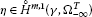and $η t (x,t)=u(x,t)$, $−∞≤t≤b$.

From the definition of generalized solution, we obtain

$( − 1 ) m ∑ | p | , | q | = 0 m ∫ Ω − ∞ b a p q D p η t D q η ¯ dxdt+i ∫ Ω − ∞ b | η t | 2 dxdt=0.$
(3.1)

Adding (3.1) to its complex conjugate, we discover

$( − 1 ) m ∑ | p | , | q | = 0 m ∫ Ω − ∞ b a p q ∂ ( D p η D p η ¯ ) ∂ t dxdt=0,$

$− ∑ | p | , | q | = 0 m ∫ Ω − ∞ b ∂ a p q ∂ t D p η D q η ¯ d x d t = lim τ → − ∞ ∑ | p | , | q | = 0 m ∫ b τ ∫ Ω a p q ( x , τ ) D p u ( x , τ ) D q u ( x , τ ) ¯ d x d τ .$
(3.2)

Using the assumption of Theorem 3.1 and the Cauchy inequality, the left-hand sides of (3.2) can be estimated by

$∑ | p | , | q | = 0 m ∫ Ω − ∞ b ∂ a p q ∂ t D p η D q η ¯ d x d t ≤ μ 1 ∑ | p | , | q | = 0 m ∫ Ω − ∞ b | D q η D p ¯ | e 2 γ t d x d t ≤ μ 1 2 ∑ | p | , | q | = 0 m ∫ Ω − ∞ b ( | D q η | 2 e 2 γ t + | D p ¯ η | 2 e 2 γ t ) d x d t ≤ μ 1 m ⋆ ∥ η ∥ H m , 0 ( γ , Ω − ∞ b ) 2 .$

From (1.1) we have

$B(τ,η,η)= ∑ | p | , | q | = 0 m ∫ Ω a p q (x,τ) D p η(x,τ) D q η ( x , τ ) ¯ dx≥ μ 0 ∥ η ( ⋅ , τ ) ∥ H m ( Ω ) 2 ,$

which implies

$lim τ → − ∞ ∑ | p | , | q | = 0 m ∫ Ω a p q (x,τ) D p η(x,τ) D q η ( x , τ ) ¯ dx≥ μ 0 lim τ → − ∞ ∥ η ( ⋅ , τ ) ∥ H m ( Ω ) 2 .$

Thus

$lim τ → − ∞ ∥ η ( ⋅ , τ ) ∥ H m ( Ω ) 2 ≤C ∥ η ∥ H m , 0 ( γ , Ω − ∞ b ) 2 .$
(3.3)

Set

$v p (x,t)= ∫ t − ∞ D p u(x,τ)dτ,−∞≤t≤b,$

then

$D p η(x,t)= ∫ b t D p u(x,τ)dτ= v p (x,b)− v p (x,t).$

Replacing them into (3.3), noting that $lim τ → − ∞ v p (x,t)=0$, yields

$∑ | p | = 0 m ∫ Ω | v p ( x , b ) | 2 d x ≤ C ∑ | p | = 0 m ∫ Ω − ∞ b e 2 γ t | D p η ( x , t ) | 2 d x d t ≤ 2 C ∑ | p | = 0 m ∫ Ω − ∞ b e 2 γ t ( | v p ( x , b ) | 2 + | v p ( x , t ) | 2 ) d x d t ≤ C e 2 γ b ∑ | p | = 0 m ∫ Ω | v p ( x , b ) | 2 d x + C ∑ | p | = 0 m ∫ Ω − ∞ b e 2 γ t | v p ( x , t ) | 2 d x d t .$

Setting

$J(t)= ∑ | p | = 0 m ∫ Ω | v p ( x , t ) | 2 dx,$

we have

$( 1 − C e 2 γ b ) J(b)≤C ∫ − ∞ b e 2 γ t J(t)dt.$

So

$J(b)≤2C ∫ − ∞ b e 2 γ t J(t)dt,∀b∈(−∞, 1 2 γ ln 1 2 C ],$

where the positive constant C depends only on μ and $μ 0$.

Using the Gronwall-Bellman inequality, we get

So $u(x,t)=0$ almost everywhere $t∈(−∞, 1 2 γ ln 1 2 C ]$. Because of the uniqueness of the solution of the problem with initial condition (2.1)-(2.3), we imply $u 1 (x,t)= u 2 (x,t)$ almost everywhere $t∈R$. □

## 4 The existence of generalized solution

The generalized solution of problem (1.2)-(1.3) can be approximated by a sequence of solutions of problems with initial condition (2.1)-(2.3).

It is known that there is a smooth function $χ(t)$ which is equal to 1 on $[1,∞)$, is equal to 0 on $(−∞,0]$ and assumes value in $[0,1]$ on $[0;1]$ (see [, Th. 5.5] for more details). Moreover, we can suppose that all derivatives of $χ(t)$ are bounded. Let $h∈(−∞,0]$ be an integer. Setting $f h (x,t)=χ(t−h)f(x,t)$, we then get

Moreover, if $f, f t ∈ L 2 (−γ, Ω R )$, $f h , f t h ∈ L 2 (−γ, Ω R )$ and

$∥ f h ∥ L 2 ( − γ , Ω R ) 2 ≤ ∥ f ∥ L 2 ( − γ , Ω R ) 2 ,$
(4.1)
$∥ f t h ∥ L 2 ( − γ , Ω R ) 2 ≤C ( ∥ f t ∥ L 2 ( − γ , Ω R ) 2 + ∥ f ∥ L 2 ( − γ , Ω R ) 2 ) ,$
(4.2)

where the constant C is independent of f, h.

Let us consider generalized solution $u h$ and $u k$ of problems (2.1)-(2.3) in cylinders $Ω h ∞$ and $Ω k ∞$ with $f(x,t)$ is replaced by $f h (x,t)$ and $f k (x,t)$ respectively. With $h>k$, $u h$ can be understood inwith $u h (x,t)=0$, $∀k≤t≤h$.

Define $u k h (x,t)= u k (x,t)− u h (x,t)$, then $u k h (x,t)$ is the generalized solution of problem (2.1)-(2.3) in cylinder $Ω k ∞$ with $f(x,t)$ is replaced by $f k h (x,t)= f k (x,t)− f h (x,t)$. According to (2.5),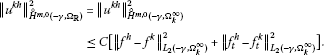Because

$∥ f h − f k ∥ L 2 ( − γ , Ω R ) 2 = ∥ f h − f k ∥ L 2 ( − γ , Ω k ∞ ) 2 = ∫ k h + 1 e − 2 γ t ∥ f h − f k ∥ L 2 ( Ω ) 2 d t = ∫ k h + 1 e − 2 γ t | χ ( t − h ) − χ ( t − k ) | ⋅ ∥ f ∥ L 2 ( Ω ) 2 d t ≤ 2 ∫ k h + 1 e − 2 γ t ∥ f ∥ L 2 ( Ω ) 2 d t .$

Because of the fact that $f∈ L 2 (−γ, Ω R )$, $lim ∫ k h + 1 e − 2 γ t ∥ f ∥ L 2 ( Ω ) 2 dt=0$ when $h,k→−∞$. So $lim ∥ f h − f k ∥ L 2 ( − γ , Ω R ) 2 =0$ when $h,k→−∞$. Repeating this argument, we discover $lim ∥ f t h − f t k ∥ L 2 ( − γ , Ω R ) 2 =0$ when $h,k→−∞$. It follows that ${ u h } h = 0 − ∞$ is a Cauchy sequence and $u h$ is convergent to u in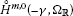.

In conclusion, we have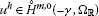satisfying

$( − 1 ) m − 1 i ∫ h T B ( t , u h , η ) dt+ ∫ Ω h T u h η t ¯ dxdt= ∫ Ω h T f h η ¯ dxdt$
(4.3)

for all $T>0$,, $η(x,t)=0$ with $t≥T$.

Because of the fact that $u h (x,t)=0$, $f h (x,t)=0$, $∀t≤h$, (4.3) leads to

$( − 1 ) m − 1 i ∫ − ∞ T B ( t , u h , η ) dt+ ∫ Ω − ∞ T u h η t ¯ dxdt= ∫ Ω − ∞ T f η ¯ dxdt$
(4.4)

for all $T>0$,, $η(x,t)=0$ for all $t≥T$.

For $f(x,t)∈ L 2 (−γ, Ω R )$, sending $h→−∞$, (4.4) is written as

$( − 1 ) m − 1 i ∫ − ∞ T B(t,u,η)dt+ ∫ Ω − ∞ T u η t ¯ dxdt= ∫ Ω − ∞ T f η ¯ dxdt$
(4.5)

for all $T>0$,, $η(x,t)=0$ for all $t≥T$.

That means $u(x,t)$ is a generalized solution of problem (1.2)-(1.3). We obtain our main result.

Theorem 4.1 Assume that:

1. (i)

$sup{| ∂ a p q ∂ t |:(x,t)∈ Ω R ,0≤|p|,|q|≤m}=μ<∞$;

2. (ii)

$| ∂ a p q ∂ t |≤ μ 1 ⋅ e 2 γ t$, for all $(x,t)∈ Ω R$, $0≤|p|,|q|≤m$;

3. (iii)

$f, f t ∈ L 2 (−γ, Ω R )$.

Then, for all $γ> γ 0 = m ⋆ μ 2 μ 0$, $m ⋆ = ∑ | α | ≤ m 1$, there exists a uniquely generalized solution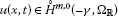of problem (1.2)-(1.3) satisfying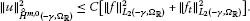## References

1. 1.

Hung NM, Anh CT: Asymptotic expansions of solutions of the first initial boundary value problem for the Schrodinger system near conical points of the boundary. Differ. Uravn. 2010, 46(2):285–289.

2. 2.

Hung NM, Anh NT: Regularity of solutions of initial-boundary value problems for parabolic equations in domains with conical points. J. Differ. Equ. 2008, 245(7):1801–1818. 10.1016/j.jde.2008.07.011

3. 3.

Bokalo NM: Problem without initial conditions for some classes of nonlinear parabolic equations. J. Sov. Math. 1990, 51: 2291–2322. 10.1007/BF01094990

4. 4.

5. 5.

Renardy M, Rogers C: An Introduction to Partial Differential Equations. Springer, Berlin; 2004.

## Acknowledgements

This research is funded by Vietnam National Foundation for Science and Technology Development (NAFOSTED) under grant number 101.01-2011.30.

## Author information

Authors

### Corresponding author

Correspondence to Nguyen Thi Lien.

### Competing interests

The authors declare that they have no competing interests.

### Authors’ contributions

All authors studied, read and approved the final manuscript.

## Rights and permissions

Reprints and Permissions

Hung, N.M., Lien, N.T. On the solvability of the boundary value problem without initial condition for Schrödinger systems in infinite cylinders. Bound Value Probl 2013, 156 (2013). https://doi.org/10.1186/1687-2770-2013-156